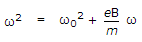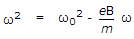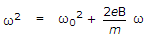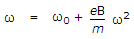# Electronics and Communication Engineering - Materials and Components

### Exercise :: Materials and Components - Section 3

41.

In carbon resistor colour code, if the third band is of silver, it indicates

 A. that three zeros are to be added to indicate the resistance value B. multiplier is 0.01 C. multiplier is 0.1 D. tolerance is ± 5%

Explanation:

No answer description available for this question. Let us discuss.

42.

In a metal, the drift velocity of electrons in the presence of a electric field E is

 A. proportional to E B. proportional to E C. inversely proportional to E D. proportional to E2

Explanation:

Drift velocity is proportional to field strength.

43.

At a temperature of about 1 K, aluminium is a

 A. conductor B. insulator C. photoconductor D. superconductor

Explanation:

No answer description available for this question. Let us discuss.

44.

An electron is moving in a circular orbit of radius R at angular velocity ω0 in a field free space. If a magnetic field B is created, the velocity of electron ω will be given by the equation

 A.B.C.D.Explanation:

No answer description available for this question. Let us discuss.

45.

If v is the velocity of electron, tc is the average time between collisions, the mean free path λ = vtc.

 A. True B. False